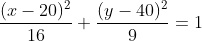## Ellipse

What is the eccentricity of the conic section represented by the below equation?Hint
The equation for an ellipse has the below format:
$$\frac{(x-h)^2}{a^2}+\frac{(y-k)^2}{b^2}=1$$$Hint 2 Eccentricity: $$e=\sqrt{1-(b^2/a^2)}$$$
A conic section is a curve obtained from the intersection of a cone’s surface and a flat plane. The eccentricity, $$e$$ , of a conic section indicates how close its shape is a circle. As eccentricity grows larger, the less the shape resembles a circle. The problem’s equation is an ellipse because it has the below format:
$$\frac{(x-h)^2}{a^2}+\frac{(y-k)^2}{b^2}=1$$$The eccentricity, $$e$$ , of a conic section indicates how close its shape is a circle. To solve for eccentricity: $$e=\sqrt{1-(b^2/a^2)}$$$
Thus,
$$e=\sqrt{1-(9/16)}=\sqrt{1-0.5625}=\sqrt{0.4375}=0.66$$\$
0.66